# Find the Largest n Values in Excel & Google Sheets

This tutorial will teach you how to find the largest n values using the LARGE function in Excel and Google Sheets.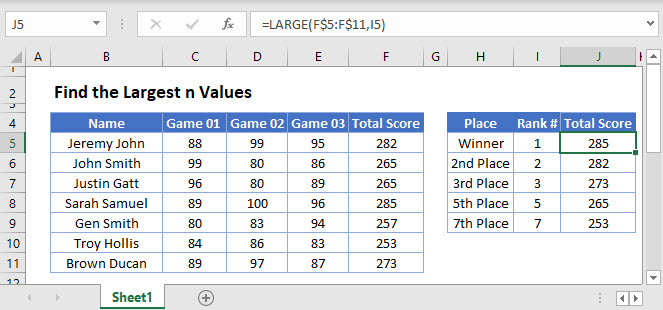## Largest n Values using LARGE Function

The LARGE function returns the nth largest numeric value from a given range (for e.g. 2nd largest, 3rd largest etc.). Its syntax is: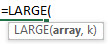The example below will return the 1st and 3rd largest values from a list.

``=LARGE(C3:C7,E3)``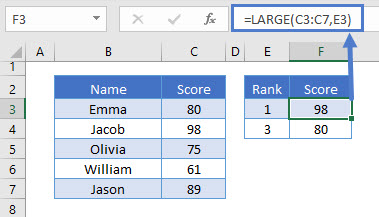As shown, the value for k (herein referred as ‘n’) finds the nth largest value

## LARGE Function using Cell Reference

In the previous example, we had to modify the formula for each respective nth position. Alternatively, an easier way is to use mixed cell reference and drag it down.

``=LARGE(C\$3:C\$7,\$E3)``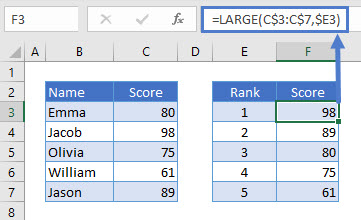Note: If the nth position is greater than the number of records in the range or if the range is empty, the LARGE function returns #NUM! error.

## LARGE Function – #NUM! Error

As noted in the previous example, #NUM! error is returned if nth position is greater than the number of records.

``=LARGE(C3:C7,6)``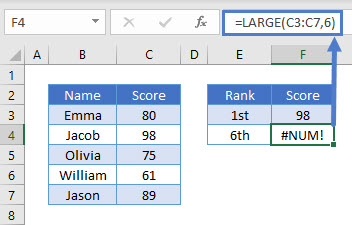## Google Sheets –Find the Largest n Values

All of the above examples work exactly the same in Google Sheets as in Excel.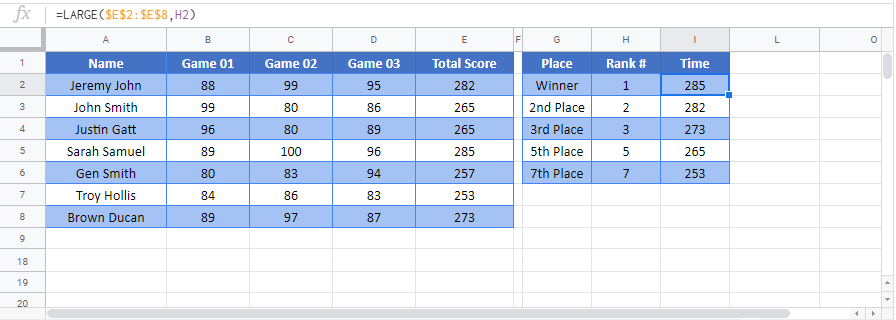### Excel Practice Worksheet

Practice Excel functions and formulas with our 100% free practice worksheets!